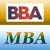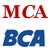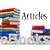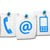Simplex Method Introduction

In the previous chapter, we discussed about the graphical method for solving linear programming problems (LPP). Although the graphical method is an invaluable aid to understand the properties of linear programming models, it provides very little help in handling practical problems.

In this chapter, we concentrate on the simplex method for solving linear programming problems with a larger number of variables.

Many different methods have been proposed to solve linear programming problems, but simplex method has proved to be the most effective. This technique will nurture your insight needed for a sound understanding of several approaches to other programming models, which will be studied in subsequent chapters. Simplex Method is applicable to any problem that can be formulated in terms of linear objective function, subject to a set of linear constraints. Often, this method is termed Dantzig's simplex method, in honour of the mathematician who devised the approach.

In the following section, we introduce you to the standard vocabulary of the simplex method.

Basic Terminology - Simplex Method Linear Programming (LP)

Slack variable

It is a variable that is added to the left-hand side of a less than or equal to type constraint to convert the constraint into an equality. In economic terms, slack variables represent left-over or unused capacity.

Specifically:
ai1x1 + ai2x2 + ai3x3 + .........+ ainxn ≤ bi can be written as
ai1x1 + ai2x2 + ai3x3 + .........+ ainxn + si = bi

Where i = 1, 2, ..., m

Surplus variable

It is a variable subtracted from the left-hand side of a greater than or equal to type constraint to convert the constraint into an equality. It is also known as negative slack variable. In economic terms, surplus variables represent overfulfillment of the requirement.

Specifically:
ai1x1 + ai2x2 + ai3x3 + .........+ ainxn ≥ bi can be written as
ai1x1 + ai2x2 + ai3x3 + .........+ ainxn - si = bi

Where i = 1, 2, ..., m

Artificial variable

It is a non negative variable introduced to facilitate the computation of an initial basic feasible solution. In other words, a variable added to the left-hand side of a greater than or equal to type constraint to convert the constraint into an equality is called an artificial variable.

Share and Recommend

•MBA & BBA
•BCA & MCA
•Ebooks & Articles
•Contact us